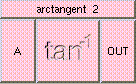ARCTANGENTTHE BOX'S MAIN HELP: This fucntion returns a scalar between [-PI/2,PI/2], the arctangent of A. Arctangent is the inverse of the tangent function where the atan(x) = y if and only if tan(y) = x. A (input): This may be any real number. OUT (output): This fucntion returns a scalar between [-PI/2,PI/2], the arctangent of A. Arctangent is the inverse of the tangent function where the atan(x) = y if and only if tan(y) = x.

<-- BACK TO Math